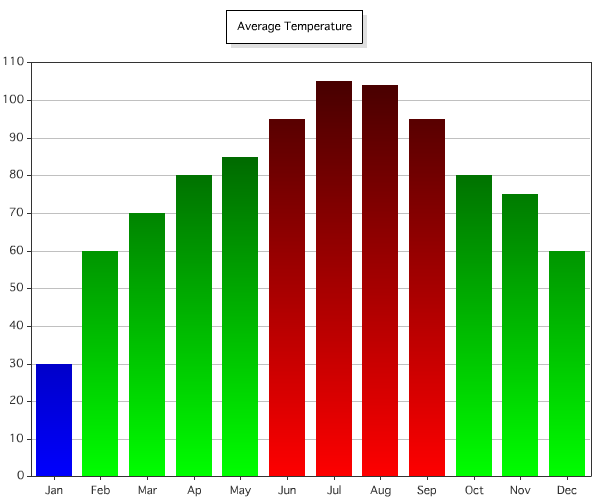Here is a question that came up earlier this morning on a mailing list. How can we specify colors for CFCHART based on some particular business rule? So for example, imagine we have a set of average temperatures. You want to chart them and specifically call out values that are below, or above, certain notes. This is rather simple if you make use of the colorList attribute of cfchartseries. Normally this is used to provide a static list of colors, but you can certainly make it dynamic as well. Here is a simple example.

Let's start off with some simple, static data, and no, this isn't real temperatures for Louisiana.

`<cfset data = arrayNew(2)> <cfset data = "Jan"> <cfset data = 30> <cfset data = "Feb"> <cfset data = 60> <cfset data = "Mar"> <cfset data = 70> <cfset data = "Ap"> <cfset data = 80> <cfset data = "May"> <cfset data = 85> <cfset data = "Jun"> <cfset data = 95> <cfset data = "Jul"> <cfset data = 105> <cfset data = "Aug"> <cfset data = 104> <cfset data = "Sep"> <cfset data = 95> <cfset data = "Oct"> <cfset data = 80> <cfset data = "Nov"> <cfset data = 75> <cfset data = "Dec"> <cfset data = 60>`

Next, let's supply this to a cfchart just to see how it looks without any modification:

`<cfchart chartheight="500" chartwidth="600" title="Average Temperature" show3d="false" > <cfchartseries type="bar" paintstyle="shade"> <cfloop index="datum" array="#data#"> <cfchartdata item="#datum#" value="#datum#"> </cfloop> </cfchartseries> </cfchart>`

This results in the rather lovely chart you see here:Ok, so it works. I'm not exactly sure where the Halloween Orange theme came from, but it works. So now let's make it dynamic. I'm going to use the following rules for my colors:

• If the temperature was less than 40, use blue (get it?).
• If the temperature was greater than 90, use red (clever, I know).
• Otherwise just use green.

I whipped up the following to generate a color list:

`<cfset cList = ""> <cfloop index="datum" array="#data#"> <cfif datum lt 40> <cfset cList = listAppend(cList, "##0000FF")> <cfelseif datum gt 90> <cfset cList = listAppend(cList, "##FF0000")> <cfelse> <cfset cList = listAppend(cList, "##00FF00")> </cfif> </cfloop>`

Next I passed in cList:

`<cfchartseries type="bar" colorlist="#cList#" paintstyle="shade">`

And voila:Not exactly a work of art, but better. You can quickly see which items are at the extreme of my data set. I've pasted the complete template below.

`<cfset data = arrayNew(2)> <cfset data = "Jan"> <cfset data = 30> <cfset data = "Feb"> <cfset data = 60> <cfset data = "Mar"> <cfset data = 70> <cfset data = "Ap"> <cfset data = 80> <cfset data = "May"> <cfset data = 85> <cfset data = "Jun"> <cfset data = 95> <cfset data = "Jul"> <cfset data = 105> <cfset data = "Aug"> <cfset data = 104> <cfset data = "Sep"> <cfset data = 95> <cfset data = "Oct"> <cfset data = 80> <cfset data = "Nov"> <cfset data = 75> <cfset data = "Dec"> <cfset data = 60>`

`<cfset cList = ""> <cfloop index="datum" array="#data#"> <cfif datum lt 40> <cfset cList = listAppend(cList, "##0000FF")> <cfelseif datum gt 90> <cfset cList = listAppend(cList, "##FF0000")> <cfelse> <cfset cList = listAppend(cList, "##00FF00")> </cfif> </cfloop>`

`<cfchart chartheight="500" chartwidth="600" title="Average Temperature" show3d="false" > <cfchartseries type="bar" colorlist="#cList#" paintstyle="shade"> <cfloop index="datum" array="#data#"> <cfchartdata item="#datum#" value="#datum#"> </cfloop> </cfchartseries> </cfchart>`